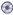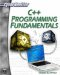C++ provides you with a powerful tool—overloading. You can overload a function so that the same name is shared by more than one function, but each function performs a different action. Function overloading is essentially when you have more than one function with the same name, but each performs different arguments. The first question that comes to your mind is probably: Why would you want to overload a function? There are several cases in which you may want to do this. For example, if you wanted to do an operation on some input, but the input came in different forms. The input might be an integer value or a floating point decimal value. You could overload the function in question, and have one version take an integer argument and the other a float argument. Let’s take a second look at our number cubed example to illustrate this.

### Example 4.3

Step 1: Open your favorite text editor and type in the following code. Save the code as04-03.cpp.

`// Include statements #include <iostream>  using namespace std; // function prototypes. float cube_number(float num); int cube_number(int num); int main() {  float number;  float number4;    cout << "Please enter a number \n";  cin >> number;  number4 = cube_number(number);  cout << number << " cubed is " << number4;  return 0;  } int cube_number(int num) {  int answer;  answer = num * num * num;  return answer; } float cube_number(float num) {  float answer;  answer = num * num * num;  return answer; }`

Step 3: Execute the code. You should see something like the image shown in Figure 4.3.Notice that there are two functions named cube_number. They both take a single argument and return that argument cubed. However, the first function takes an integer and returns an integer answer, whereas the second function takes a floating point number and returns a floating point answer. The big question is how does the compiler know which function you are calling? The answer is simple, it knows which function you are calling based on what kind of argument you pass it. If you pass an argument with a decimal point, then it will call the function that takes a floating decimal point. If you pass an argument without a decimal point, then it will call the function that takes an integer.

This is why all overloaded functions MUST have different parameter types and/or a different number of parameters. You will see examples with different numbers of parameters later in this book.C++ Programming Fundamentals (Cyberrookies)
ISBN: 1584502371
EAN: 2147483647
Year: 2005
Pages: 197
Authors: Chuck Easttom